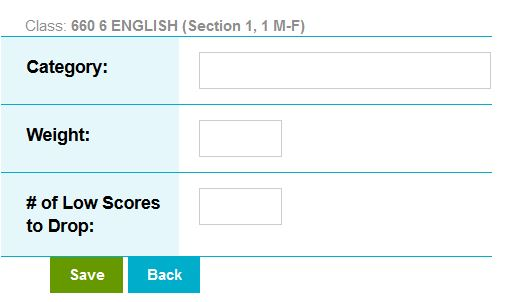# How do I create a gradebook category?

Categories are used to organize assignments in the gradebook and to assign weights to graded work.

To create categories in the gradebook:

1. Go to Instructor Main > Gradebook > Setup > Basic Setup > Categories
2. Select Create Category
3. Enter the category information:
• Category:  Enter the name of the category (ie. Quiz, Homework, etc.)
• Weight:  Enter a numeric value that represents the weight that the category will have in the gradebook average calculation (ie. 50)
• # of Low Scores to Drop:  Enter the number of lowest scored assignments in a category to not be included in the grade calculationMost commonly, the total of all categories will equal 100.  When the total weight of all categories equals 100, each category represents a percentage of the total grade.

Was this article helpful?
0 out of 0 found this helpful
Have more questions? Submit a request